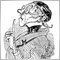# loop comparison of EMA values44

Hello, i want to write my function so that it makes a for loop comparison of different EMAs for order execution. In theory a for loop would go through all the numbers, but mine does nothing. Any ideas?

```void CheckForEMATrade(){
int x,y;
int period[]={20,25,30,35,40,45,50,55,60,65,70};
double EMAx=iMA(Symbol(),0,period[x],0,MODE_EMA,PRICE_CLOSE,1),
EMAy=iMA(Symbol(),0,period[y],0,MODE_EMA,PRICE_CLOSE,1);

for(x=0;x<10;x++)
for(y=1;y<=10;y++){
if(EMAy<EMAx)printf("sell");

}

}
```Moderator
12453

```void CheckForEMATrade(){
int x,y;
int period[]={20,25,30,35,40,45,50,55,60,65,70}; // size=11!
for(x=0;x<10;x++)
for(y=1;y<=10;y++){
double EMAx=iMA(Symbol(),0,period[x],0,MODE_EMA,PRICE_CLOSE,1),
EMAy=iMA(Symbol(),0,period[y],0,MODE_EMA,PRICE_CLOSE,1);
if(EMAy<EMAx)printf("sell");
}

}```

but maybe y should always be smaller than x?

```for(x=1;x<11;x++)
for(y=0;y<x;y++){```44

gooly:

but maybe y should always be smaller than x?

As far as i know array numeration is starting from 0!
BUT! thanks! in fact for loops need variables to be embedded and not being declared outside, that was the problem actually. :)Moderator
31751

trisperon:
As far as i know array numeration is starting from 0!
BUT! thanks! in fact for loops need variables to be embedded and not being declared outside, that was the problem actually. :)

It is not clear from your reply whether you are agreeing or disagreeing with Gooly.

Gooly's suggestion is correct as far as I can see.

Maybe it would be a good idea for you to post your amended code.